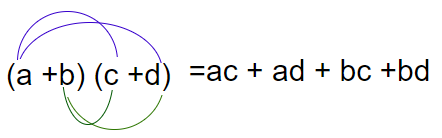# Class 8 maths exercise 9.4 solutions

In this page we have Class 8 maths exercise 9.4 solutions for Algebraic Expressions .This exercise has questions about multiplication of polynomials by polynomials. Hope you like them and do not forget to like , social share and comment at the end of the page.

## Class 8 maths exercise 9.4 solutions

Question 1
Multiply the binomials.
(i) (2x + 5) and (4x – 3)
(ii) (y – 8) and (3y – 4)
(iii) (2.5l – 0.5m) and (2.5l + 0.5m)
(iv) (a + 3b) and (x + 5)
(v) (2pq + 3q2) and (3pq – 2q2)
Let  ( a+b) (c+d) to be done
then
(a+b) (c+d)= a(c+d) + b( c+d)
=a x  c+a x  d+b x  c+b x dWe will use the same concept in all the question below
(i) (2x + 5)(4x - 3)
2x x 4x - 2x x 3 + 5 x 4x - 5 x 3
8x² - 6x + 20x -15
8x² + 14x -15
(ii) ( y - 8)(3y - 4)
= y x 3y - 4y - 8 x 3y + 32
= 3y2 - 4y - 24y + 32
= 3y2 - 28y + 32
(iii) (2.5l - 0.5m)(2.5l + 0.5)
Using (a+b)(a-b) = a2 - b2
We get = 6.25l2 - 0.25m2
(iv) ax + 5a + 3bx + 15b
v) 2pq x 3pq - 2pq x 2q2 + 3q2 x 3pq - 3q2 x 2q2
= 6p2q2 - 4pq3 + 9pq3 - 6q4 = 6p2q2 - 5pq3 - 6p4

Question 2
Find the product.
(i) (5 – 2x) (3 + x)
(ii) (x + 7y) (7x – y)
(iii) (a2+ b) (a + b2)
(iv) (p2– q2) (2p + q)
(i) 15 + 5x - 6x - 2x2
= 15 - x - x 2
(ii) 7x2 - xy + 49xy - 7y2
= 7x2 - 7y2 + 48xy
(iii) a2 x a + ax b + a x b + b3
= a3 + a2b + ab + b3
= a3 + b3 + a2b + ab
(iv) 2p3 + p2q - 2pq2 - q3
= 2p3 - q3 + p2q - 2pq2

Question 3
Simplify.
(i) (x2– 5) (x + 5) + 25
(ii) (a2+ 5) (b3+ 3) + 5
(iii) (a + b) (c – d) + (a – b) (c + d) + 2 (ac + bd)
(iv) (x + y)(2x + y) + (x + 2y)(x – y)
(v) (x + y)(x2– xy + y2)
(vi) (1.5x – 4y)(1.5x + 4y + 3) – 4.5x + 12y
(vii) (a + b + c)(a + b – c)
(i) x3 + 5x2 - 5x - 25 + 25
= x3 + 5x2 -5x
(ii) a2b3 + 3a2 + 5b3 + 15 + 5
= a2b3 + 5b3 + 3a2 + 20
(iii) (ac - ad + bc - bd) + (ac + ad - bc - bd) + (2ac + 2bd)
= ac - ad + bc - bd + ac + ad - bc - bd + 2ac + 2bd
= 4ac
(iv) 2x2 + xy + 2xy + y2 + x2 - xy + 2xy - 2y2
= 3x2 + 4xy - y2
(v) x3 - x2y + xy2 + x2y - xy2 + y3
= x3 + y3
(vi) 2.25x2 + 6xy + 4.5x - 6xy - 16y2 - 12y - 4.5x + 12y
= 2.25x2 - 16y2
(vii) a2 + ab - ac + ab + b2 - bc + ac + bc - c2
= a2 + b2 - c2 + 2ab

## Summary

1. Class 8 maths exercise 9.4 solutions has been prepared by Expert with utmost care. If you find any mistake.Please do provide feedback on mail. You can download the solutions as PDF in the below Link also
2. This chapter 9 has total 5 Exercise 9.1 ,9.2 ,9.3 ,9.4 and 9.5. This is the Fourth exercise in the chapter.You can explore previous exercise of this chapter by clicking the link below